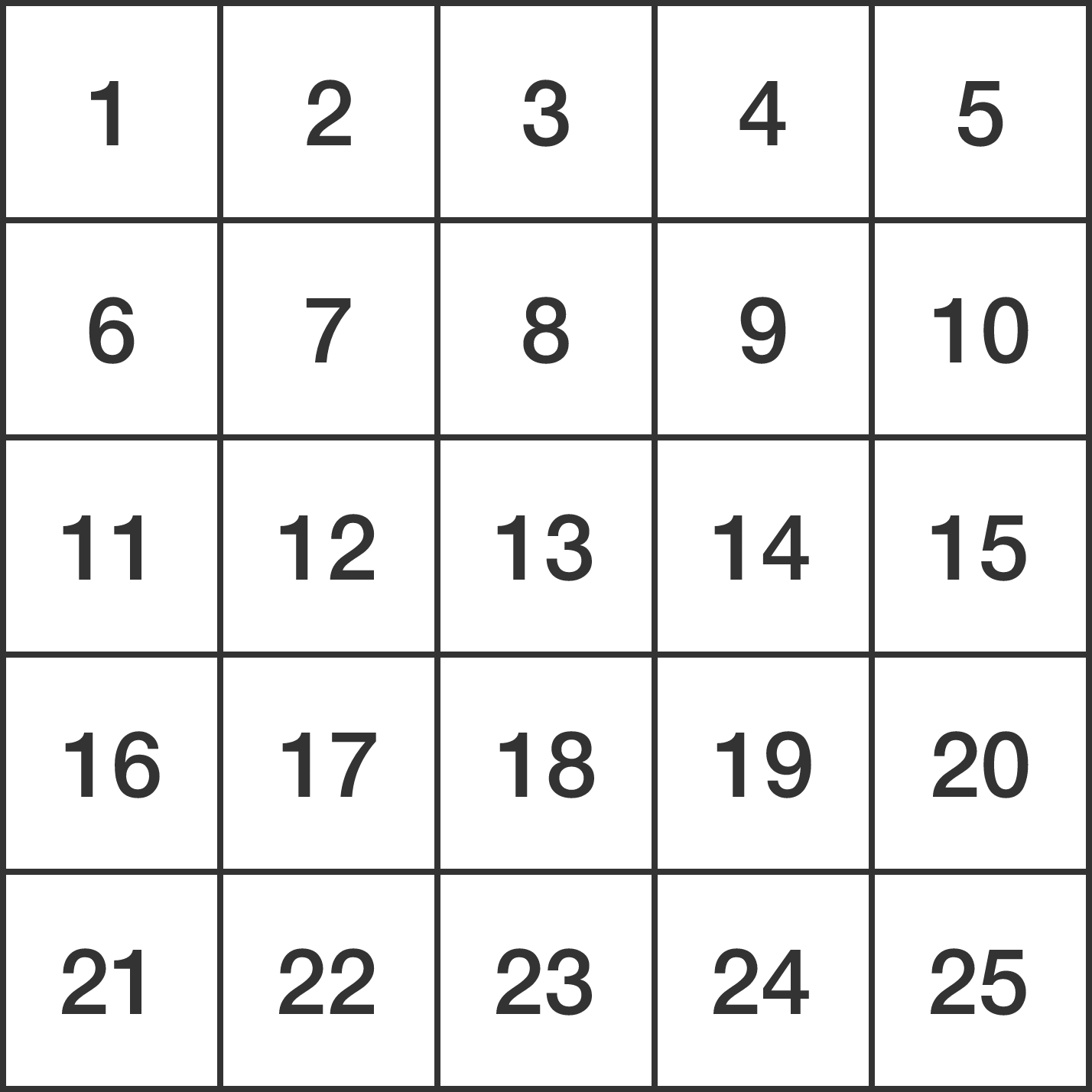# Keep The Difference Small

Probability Level 4

Filling a $5 \times 5$ grid with distinct integers from 1 to 25, what is the minimum of the maximum of the differences between 2 squares that share a common side?


Hint: As an explicit example, for the naive arrangement belowthe maximum of the differences between 2 squares sharing a common side is equal to 5. This is because, in the above table which is one of all $25!$ possible arrangements, the maximum never exceeds 5, as can be seen by the differences between many neighboring cells: $6-1=7-2=\cdots=24-19=25-20,$ which are all equal to 5. Hence, the minimum of the maximum we are looking for is at most 5.

×